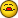removing #na from a sheet

E

excelquester

how do i remove the # na from showing up in cells where formulas are required
on the sheet ,, how ever there is no information required in the lookup_value
cell.
using the formula =VLOOKUP(A2,Sheet1!A,2,FALSE)
Example:

Col a Col b col c
row 2 A1011 name special information
row 3 A1012 name 0
row 4 A1013 name 0

row 6 blank #na #na
row 7 blank #na #na

J

Jacob Skaria

Handle that using ISNA() and IF() ..If you are using XL2007 you can try
IFERROR()

=IF(ISNA(VLOOKUP(A2,Sheet1!A,2,0)),"",VLOOKUP(A2,Sheet1!A,2,0))

If this post helps click Yes

M

T

T. Valko

One way:

=IF(ISNA(VLOOKUP(A2,Sheet1!A,2,0)),"",VLOOKUP(A2,Sheet1!A,2,0))

E

Eduardo

Hi,

=if(ISNA(=VLOOKUP(A2,Sheet1!A,2,FALSE)),"",=VLOOKUP(A2,Sheet1!A,2,FALSE))

E

excelquester

getting a error,, something about using = , and - in a formula

B

Bob I

remove the extraneous = signs

J

Jacob Skaria

Remove the = sign from the formulas..or check the other posts

If this post helps click Yes